#DAY 5
0
AI & Data

## AI & Data_5(Perceptron)

Perceptron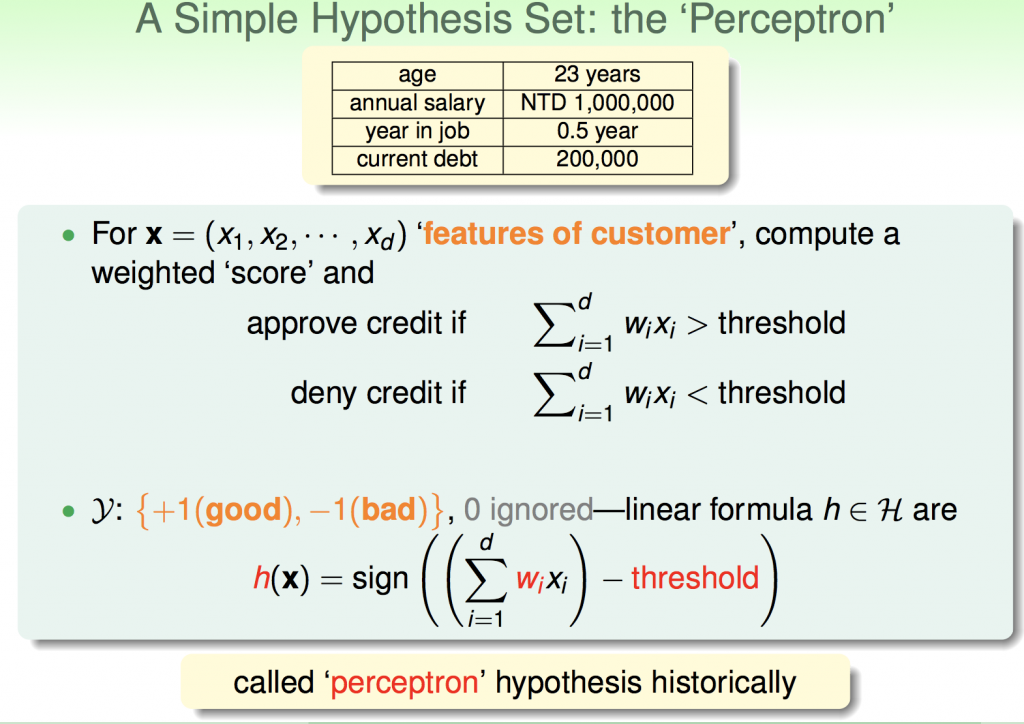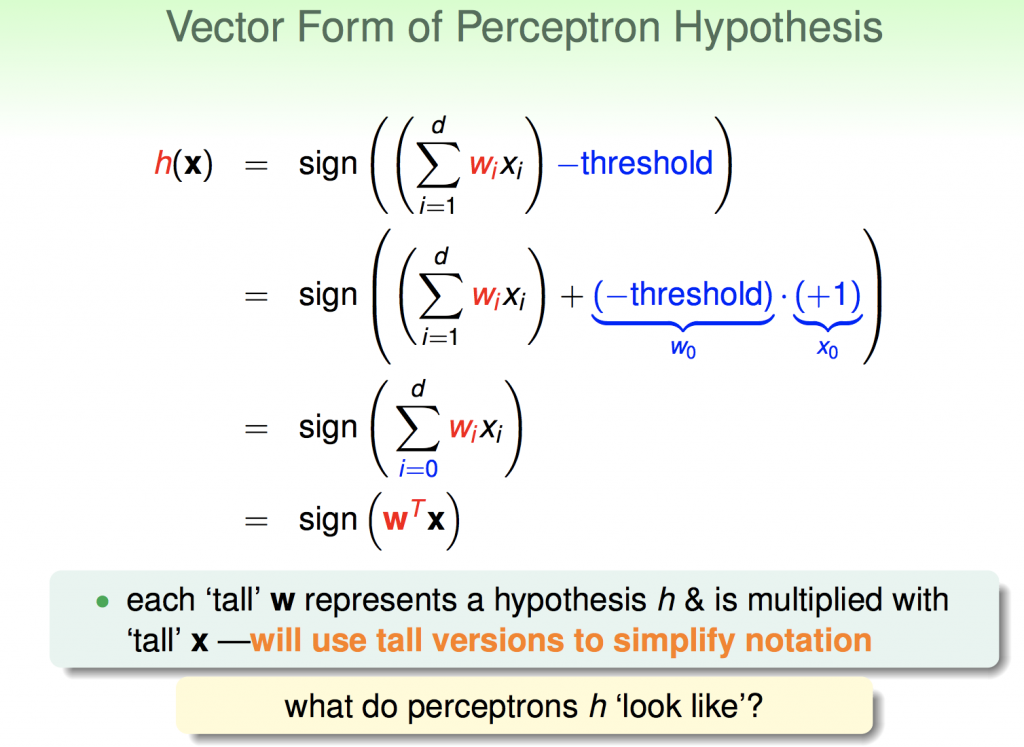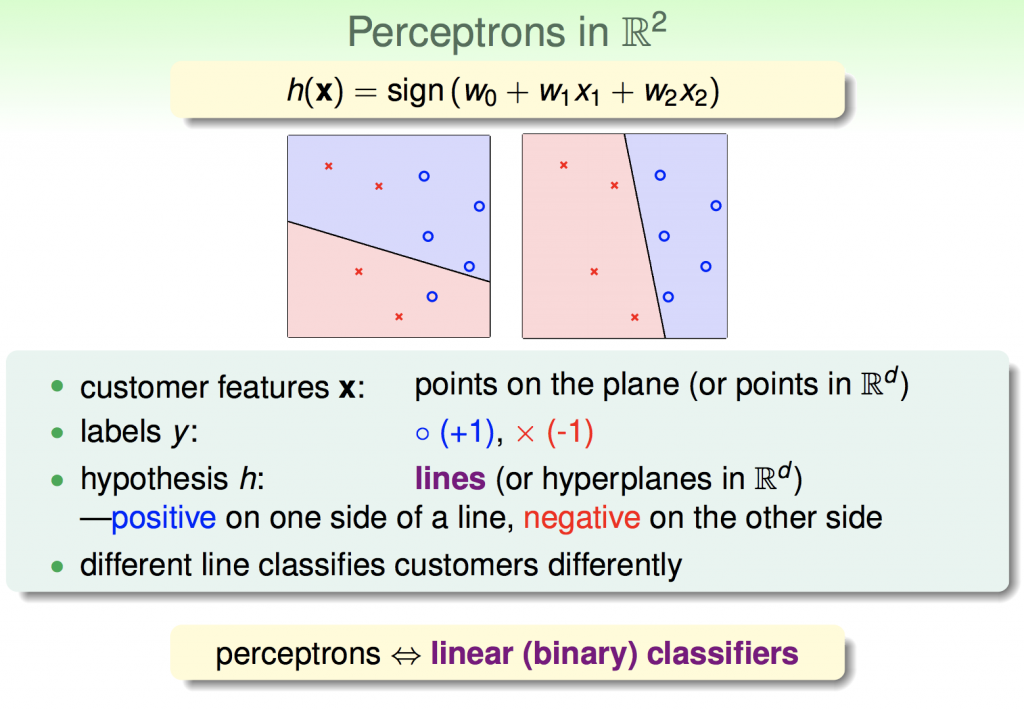Perceptron Learning Algorithm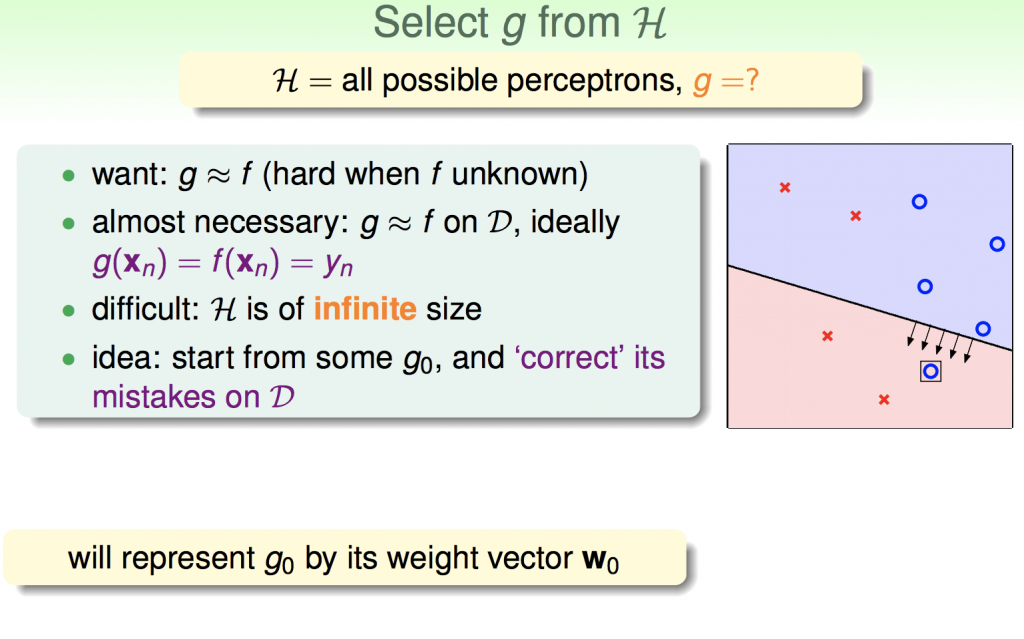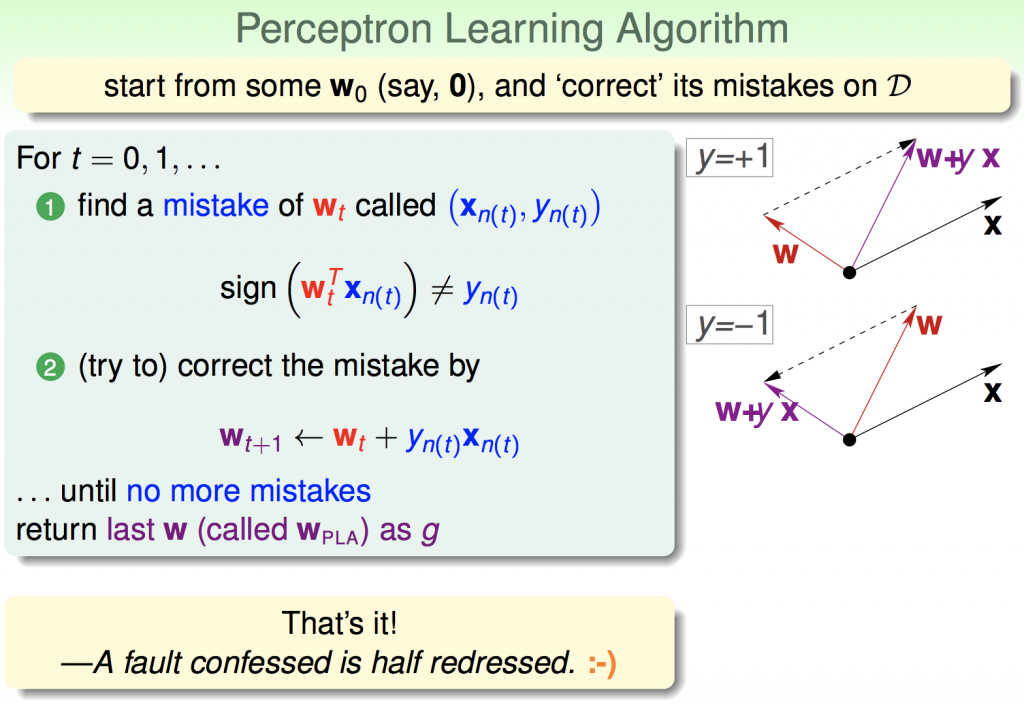``````import numpy as np
import pandas as pd
import matplotlib.pyplot as plt

class Perceptron:
def __init__(self, eta=0.01, n_iter=10):
self.eta = eta
self.n_iter = n_iter

def fit(self, X, y):
self.w = np.zeros(1 + X.shape)
self.errors = []

for _ in range(self.n_iter):
error = 0
for xi, target in zip(X, y):
update = self.eta * (target - self.predict(xi))
self.w[1:] += update * xi
self.w += update
error += int(update != 0.0)
self.errors.append(error)
return self

def net_input(self, X):
return np.dot(X, self.w[1:]) + self.w

def predict(self, X):
return np.where(self.net_input(X) >= 0.0, 1, -1)

def main():
X = df.iloc[0:100, [0, 2]].values
y = df.iloc[0:100, 4].values
y = np.where(y == 'Iris-setosa', -1, 1)

plt.scatter(X[:50, 0], X[:50, 1], color='red', marker='o', label='setosa')
plt.scatter(
X[50:100, 0],
X[50:100, 1],
color='blue',
marker='x',
label='versicolor')
plt.xlabel('petal length')
plt.ylabel('sepal length')
plt.legend(loc='upper left')
plt.show()

if __name__ == '__main__':
main()
``````